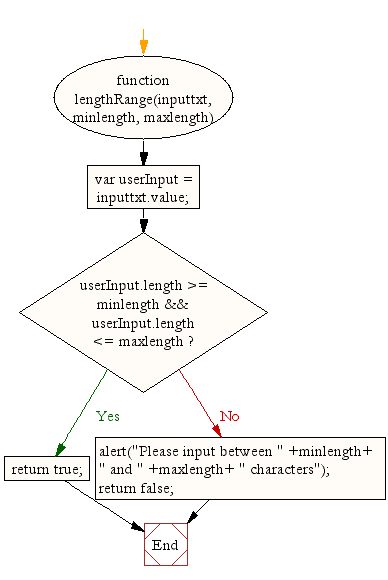# JavaScript: HTML Form - restricting the length

## Checking string length

Sometimes situation arises when a field in an html form accept a restricted number of characters. For example a userid (length between 6 to 10 character) or password (length between 8 to 14 characters). You can write a JavaScript form validation script where the required field(s) in the HTML form accepts the restricted number of characters.

Javascript function to restrict length of user input

``````function lengthRange(inputtxt, minlength, maxlength)
{
var userInput = inputtxt.value;
if(userInput.length >= minlength && userInput.length <= maxlength)
{
return true;
}
else
{
alert("Please input between " +minlength+ " and " +maxlength+ " characters");
return false;
}
}
```
```

Flowchart:Here's an example of the above function for a field that requires 6 to 8 characters to valid a usercode.

HTML Code

``````<!DOCTYPE html>
<html lang="en">
<head>
<meta charset="utf-8">
<title>JavaScript form validation - checking non empty</title>
<link rel='stylesheet' href='form-style.css' type='text/css' />
</head>
<body onload='document.form1.text1.focus()'>
<div class="mail">
<p>Enter Userid [between 6 to 8 characters] and Submit</p>
<form name="form1" action="#">
<ul>
<li>Username:<input type='text' name='text1'/></li>
<li>&nbsp;</li>
<li class="submit"it"><input type="submit" name="submit" value="Submit" onclick="stringlength(document.form1.text1,6,8)"/></li>
<li>&nbsp;</li>
</form>
</ul>
</div>
<script src="string-lenght.js"></script>
</body>
</html>
```
```

Javascript code

``````function stringlength(inputtxt, minlength, maxlength)
{
var field = inputtxt.value;
var mnlen = minlength;
var mxlen = maxlength;

if(field.length<mnlen || field.length> mxlen)
{
alert("Please input the userid between " +mnlen+ " and " +mxlen+ " characters");
return false;
}
else
{
alert('Your userid have accepted.');
return true;
}
}
```
```

CSS Code

``````li {list-style-type: none;
font-size: 16pt;
}
.mail {
margin: auto;
padding-top: 10px;
padding-bottom: 10px;
width: 400px;
background : #D8F1F8;
border: 1px soild silver;
}
.mail h2 {
margin-left: 38px;
}
input {
font-size: 20pt;
}
input:focus, textarea:focus{
background-color: lightyellow;
}
input submit {
font-size: 12pt;
}
.rq {
color: #FF0000;
font-size: 10pt;
}
```
```

View the example in the browser

Practice the example online

See the Pen string-length-1 by w3resource (@w3resource) on CodePen.

file_download Download the validation code from here.

Other JavaScript Validation:

﻿

## JavaScript: Tips of the Day

Returns the number of times a function executed per second. hz is the unit for hertz, the unit of frequency defined as one cycle per second

Example:

```const tips_hz = (fn, iterations = 100) => {
const before = performance.now();
for (let i = 0; i < iterations; i++) fn();
return (1000 * iterations) / (performance.now() - before);
};
// 10,000 element array
const numbers = Array(10000)
.fill()
.map((_, i) => i);

// Test functions with the same goal: sum up the elements in the array
const sumReduce = () => numbers.reduce((acc, n) => acc + n, 0);
const sumForLoop = () => {
let sum = 0;
for (let i = 0; i < numbers.length; i++) sum += numbers[i];
return sum;
};

// 'sumForLoop' is nearly 10 times faster
console.log(Math.round(tips_hz(sumReduce)));
console.log(Math.round(tips_hz(sumForLoop)));
```

Output:

```4004
990
```